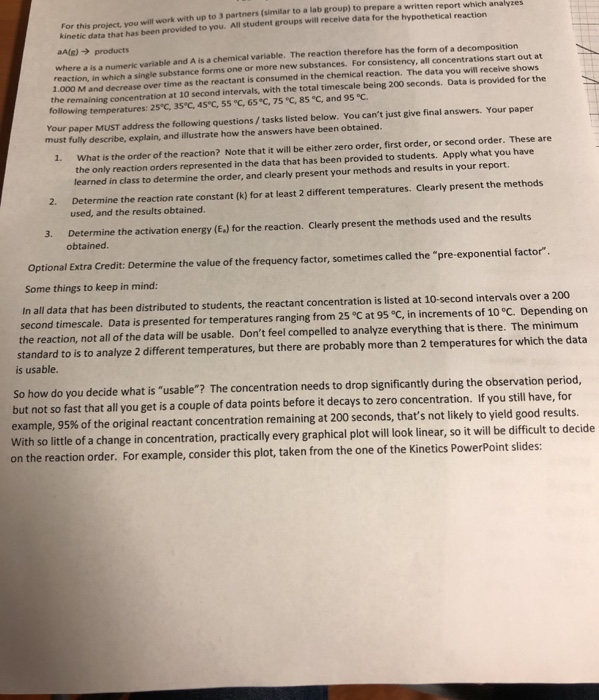Question

# For a physiology class experiment, a student is fitted with a lightweight waterproof device that measures...

For a physiology class experiment, a student is fitted with a lightweight waterproof device that measures her average pulse rate over 2-hourly intervals. Consider 12 consecutive intervals over a 24-hour period, and let X be the number of these intervals in which the average pulse rate is less than 100 beats per minute. Is it reasonable to treat X as an observation from a binomial distribution? Justify your answer

Number of trials,that is number of intervals, are fixed to 12. The number of outcomes in each trials: pulse rate is less than 100 or pulse rate is not less than 100

The probability that pulse rate will be less than 100 is not given. Logically it may change for each trial. Therefore we cannot assume that probability of success is constant for each trial.

So, it cannot be modeled as binomial distribution.

Answer: It is not reasonable to treat X as an observation from a binomial distribution.

#### Earn Coins

Coins can be redeemed for fabulous gifts.

Similar Homework Help Questions
• ### will work with up to 3 partners (similar to a lab group) to prepare a written report which analyzes kinetic data that has been provided to you. All student groups will receive data for the hy...will work with up to 3 partners (similar to a lab group) to prepare a written report which analyzes kinetic data that has been provided to you. All student groups will receive data for the hypothetical reaction aAlE) products where a is a numeric variable and A is a chemical variable. The reaction therefore has the form of a decomposition reaction, in which a single substance forms one or more new substances. For consistency, all concentrations start out at 1.000...